7.2 States of a Bistable Defect

In the eNMP model, the defects are described by the form of their adiabatic potentials. Motivated by TDDS and EPR experiments , they are assumed to feature one stable and one metastable configuration. This bistability is reflected in the double well form of their adiabatic potentials (see Fig. 7.2). Note that it is the key aspect of the eNMP model since it can give an explanation for a plenty of challenging experimental observations, addressed later in this chapter.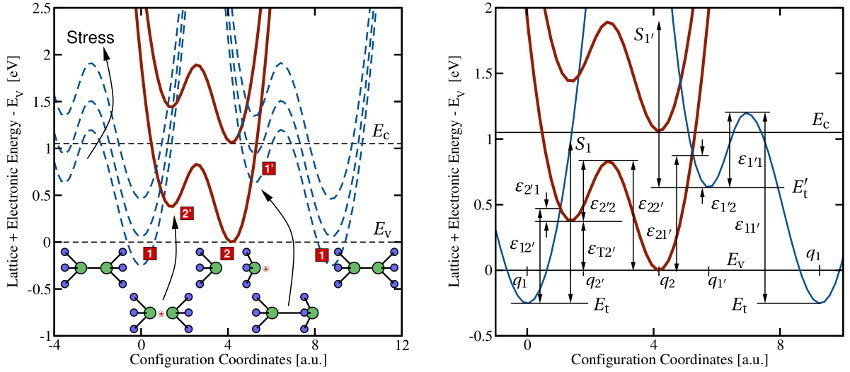Figure 7.2: Left: A schematic of the configuration coordinate diagram for a bistable defect. The solid red and the blue dashed lines represent the adiabatic potentials for a defect in its positive and neutral charge state, respectively. The energy minima correspond to the stable or metastable defect configurations, labeled bywith. The present configuration coordinate diagram describes the exchange of holes with the valence band and thus is associated with a hole capture or emission process. The stick-and-ball models display a defect in its various stable and metastable configurations. A possible candidate for such a bistable defect might be the well-known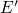center, which is frequently invoked in the context of noise in MOSFETs. Right: Definitions of the used energies and barriers in the eNMP model. Recall that two adiabatic potentials must be shown for one transition. It is assumed that an alternative transition pathway with an additional crossing point exists in the multi-dimensional atomic configuration space. In order to show both intersections (related toand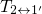) in one configuration coordinate diagram, the ‘neutral’ potential must be plotted twice. Obviously,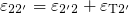and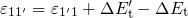hold.

The configuration coordinate diagram of such a bistable defect is depicted in Fig. 7.2. The numbersand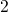denote the positive and neutral charge state of the defect, respectively, and the metastable states are marked by additional primes. In the configuration coordinate diagram, there exist two crossing points, where each of them is related to one of the two charge transfer reactions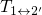and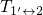. Their corresponding NMP barriers1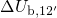and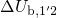are derived by evaluating equation (7.17) for the energy differences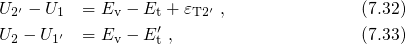respectively (see Fig. 7.2). The resulting expressions for the NMP barriers read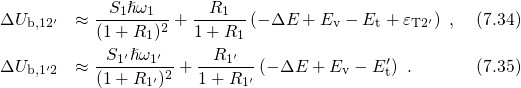Inserting them into the equations (7.30) and (7.31) delivers the transition rates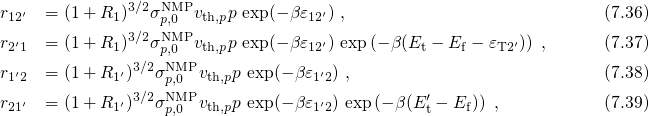with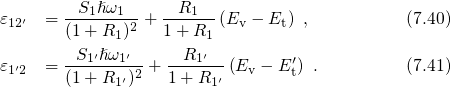In order to reduce the number of fitting parameters in the numerical simulations, the cross sections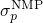are expected to be within the same order of magnitude for all charge transfer reactions and are thus set equal. The field dependence of the charge transfer reactions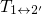and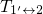is governed by the relative position of the ‘neutral’ and the ‘positive’ adiabatic potential. When a negative bias is applied to the gate of a pMOSFET (see Fig. 7.2), the ‘neutral’ potential is raised. As a result, the barriers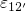and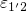are reduced, which facilitates the charge transfer reactions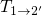and, respectively. Conversely, the transitions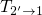and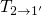are slowed down since the corresponding barrier heights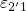and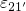have become larger. The transitionsandare thermally activated and do not vary with the applied gate bias. According to transition state theory, they can be expressed as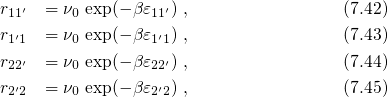where the barriers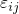are defined as in Fig. 7.2 and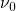stands for the attempt frequency, which is typically of the order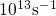. Using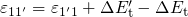and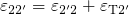, the rates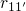and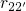can be rewritten as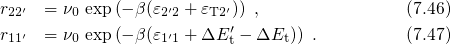The defect in the eNMP model has a state diagram as shown in Fig. 7.3. With the rates (7.36)-(7.47), the defect kinetics are described by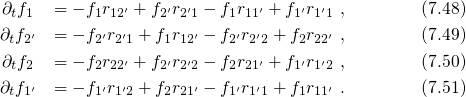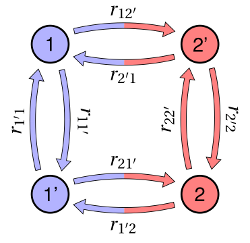Figure 7.3: The state diagram of the eNMP model. The individual states are represented by the circles, where the red and the blue color indicate the positive and the neutral charge state of the defect, respectively. The transition rates between two states are denoted by the colored arrows. The vertical transitions correspond to pure thermal transitions while horizontal ones stand for charge transfer reactions, which are detectable in NBTI, RTN, and TDDS experiments.